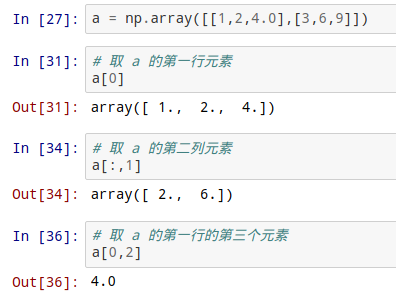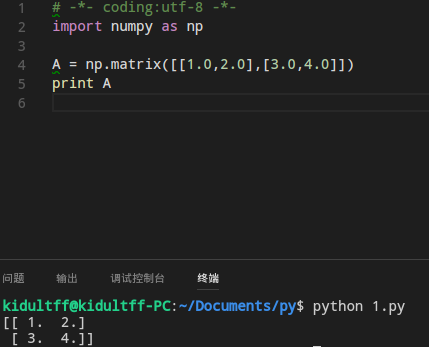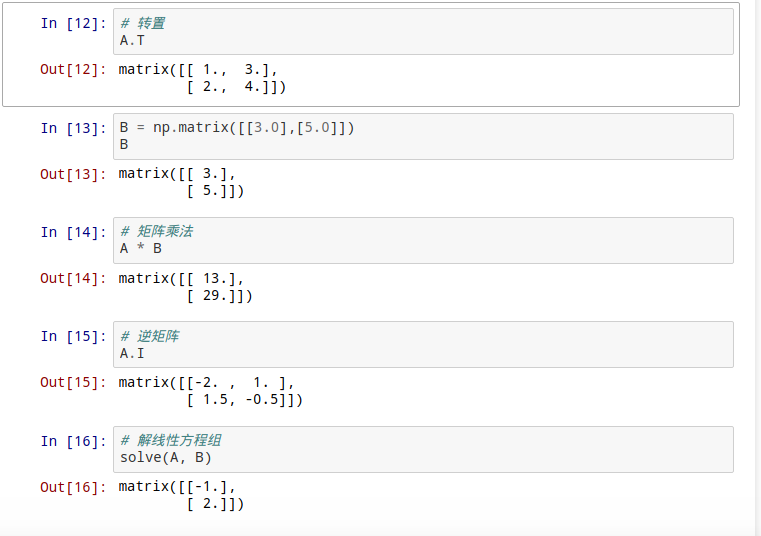# Python科学计算 - Numpy库

Numpy是高性能科学计算和数据分析的基础包。

### 一、ndarray数组基础

Python 有 array 模快，但他不支持多维数组，无论是列表还是 array 模块都没有科学运算函数，不适合做矩阵等科学计算。Numpy没有使用 Python 本身的数组机制，而是提供了 ndarray 数组对象，该对象不断能方便的存取数组，而且拥有丰富的数组计算函数，比如向量的加法、减法、乘法等。

``import numpy``

#### 1.1 创建数组

``````# -*- coding:utf-8 -*-
import numpy as np

a = np.array([[1,2,4.0],[3,6,9]]) #定义多维数组
print a

b = np.array([[1,2,4],[3,6,9]]) #定义多维数组
print b

print a.ndim    #行数
print a.shape   #数组大小
print a.dtype   #数组数据类型
print b.dtype``````#### 1.2 特殊数组

Numpy的特殊数组主要有以下几种：

• zeros数组：全零数组，元素全为0；

• ones数组：全1数组，元素全为1;

• empty数组：空数组，元素全近似为0；

``````# -*- coding:utf-8 -*-
import numpy as np

print np.zeros((2,3))   #0数组
print np.ones((2,3))    #1数组
print np.empty((2,3))   #空数组``````### 二、矩阵

#### 2.1 创建矩阵

Numpy的矩阵对象与数组对象相似，主要不同之处在于，矩阵对象的计算遵循矩阵数学运算规律。矩阵使用 matrix 函数创建，以（2,2）大小的矩阵为例（2行2列），定义方法如下：

``````# -*- coding:utf-8 -*-
import numpy as np

A = np.matrix([[1.0,2.0],[3.0,4.0]])``````